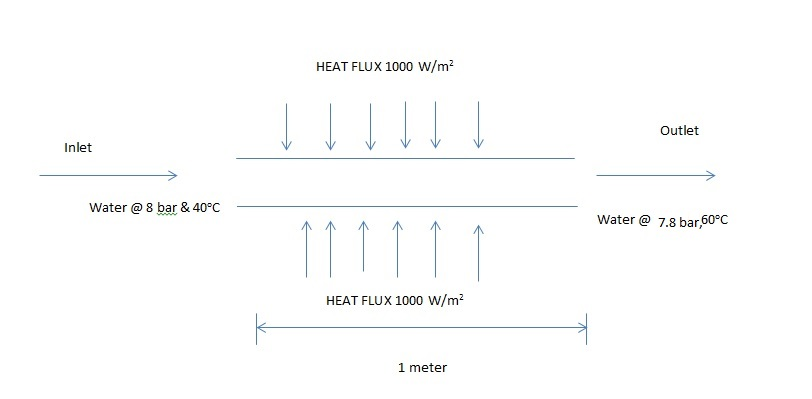# Is flow is compressible or incompressible??I have enclosed a image file which defines the flow conditions of a pipe. i have one doubt whether flow is compressible or incomprehensible??? how to write continuity equation (differential) for steady state conditions???

• edited September 2018

If you do the calculations what is the difference in density? Then compare the difference with the overall density. You may also need to factor in the flow rate, what is that?

• Hello,

Just to add to rwoolhou's answer, you should be able to get the continuity equation for this problem in any standard fluids and heat transfer textbook. Just search for internal flow and heat transfer through a pipe with constant wall flux.

Best,

Karthik

• edited September 2018

Hi,

As a first approach I would assume the flow to be incompressible. You can assess this a priori by calculating the Mach number of the flow based on the speed of sound in the water. For Mach < 0.3 assuming incompressibility is a good approximation (without buoyancy effects).

• edited September 2018

I assume the water is liquid. Please find out the water density at inlet and outlet respectively. If the density change is over 5%, the water is compressible. Otherwise it is incompressible.